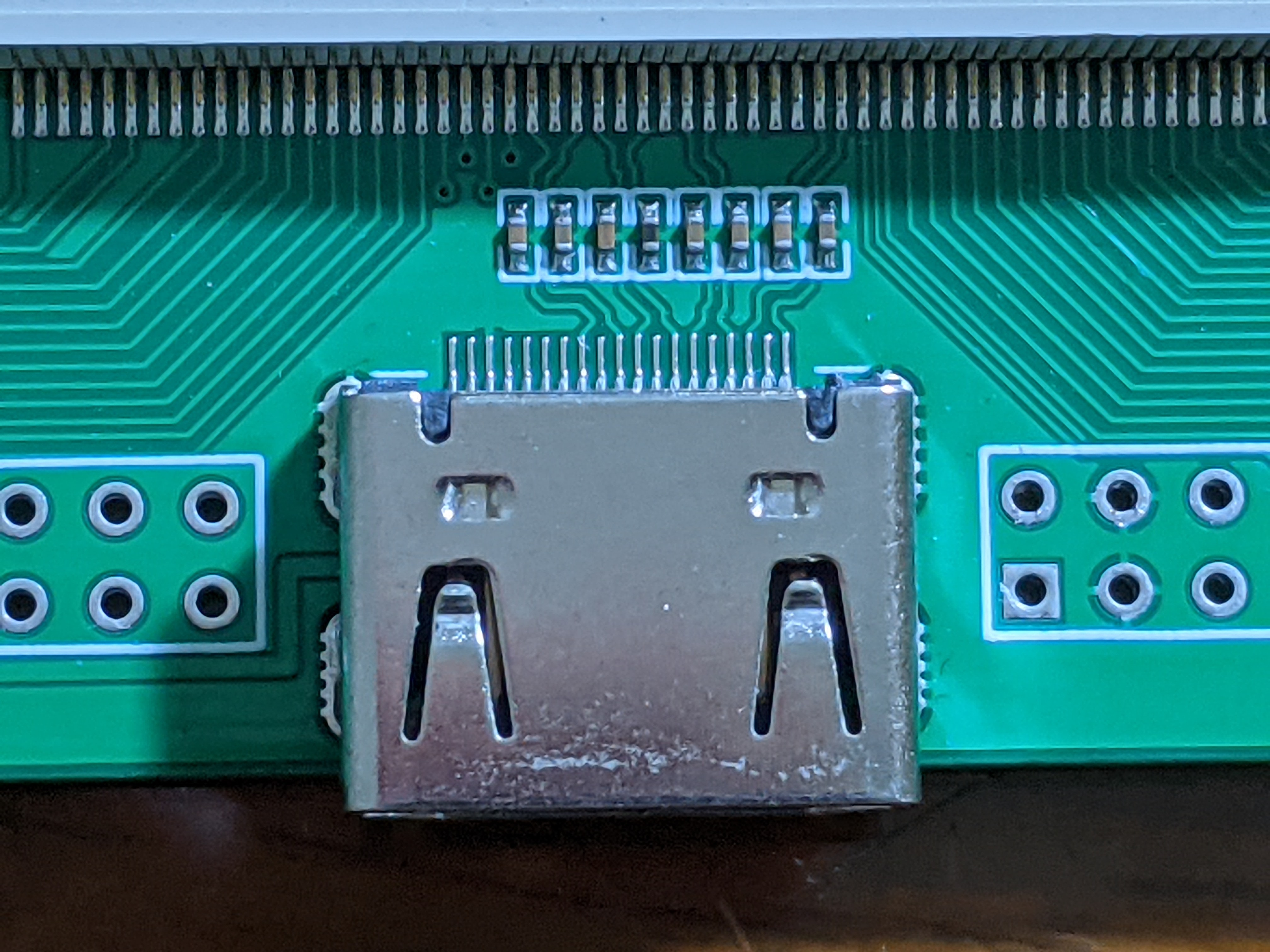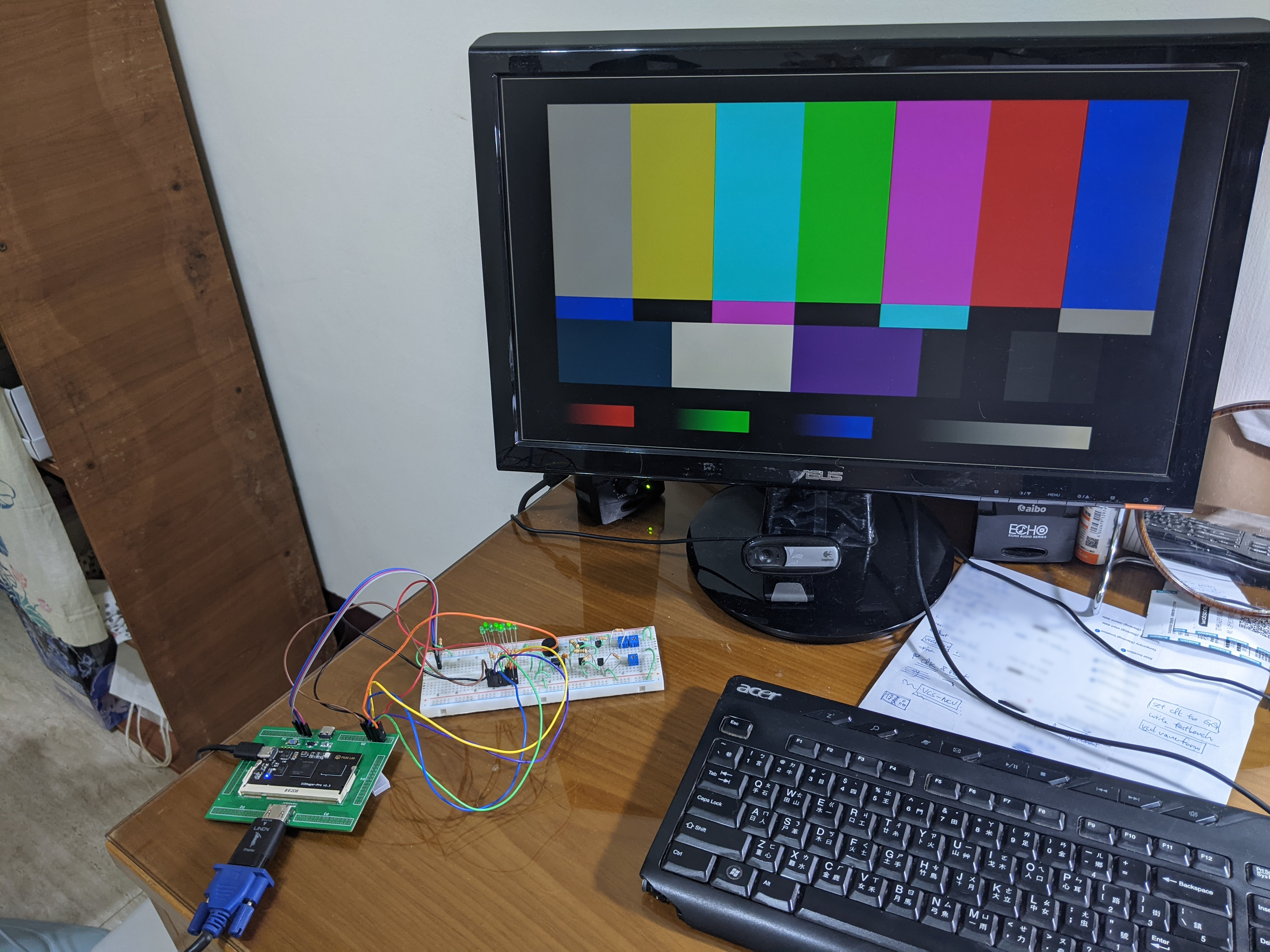# HDMI protocol#

High Definition Multimedia Interface，HDMI （高畫質多媒體介面）是應用相當廣泛的視訊介面，板子使用的是 HDMI pin A 17 支針腳 ， 不過如果近拍的話，可以看到它只連接了 8 支腳跟接地，分別是：

• 1,3 Data 2+/- 藍色
• 4,6 Data 1+/- 綠色
• 7,9 Data 0+/- 紅色
• 10,12 Data Clock +/-HDMI 的信號走的是差動傳輸，比起 UART 的單端傳輸能做到更高的速率，相關的文章可以看這篇 ， 因為沒接所以我們沒辦法玩 HDMI 後面針腳提供 I2C，反正那個應該是用來走音訊用的，不玩沒差(欸。

``````LOCATE COMP "hdmi_dp" SITE "G1"; # Blue +
LOCATE COMP "hdmi_dn" SITE "F1"; # Blue -
LOCATE COMP "hdmi_dp" SITE "J1"; # Green +
LOCATE COMP "hdmi_dn" SITE "H2"; # Green -
LOCATE COMP "hdmi_dp" SITE "L1"; # Red +
LOCATE COMP "hdmi_dn" SITE "K2"; # Red -
LOCATE COMP "hdmi_dp" SITE "E2"; # Clock +
LOCATE COMP "hdmi_dn" SITE "D3"; # Clock -

IOBUF PORT "hdmi_dp" IO_TYPE=LVCMOS33 DRIVE=4;
IOBUF PORT "hdmi_dn" IO_TYPE=LVCMOS33 DRIVE=4;
IOBUF PORT "hdmi_dp" IO_TYPE=LVCMOS33 DRIVE=4;
IOBUF PORT "hdmi_dn" IO_TYPE=LVCMOS33 DRIVE=4;
IOBUF PORT "hdmi_dp" IO_TYPE=LVCMOS33 DRIVE=4;
IOBUF PORT "hdmi_dn" IO_TYPE=LVCMOS33 DRIVE=4;
IOBUF PORT "hdmi_dp" IO_TYPE=LVCMOS33 DRIVE=4;
IOBUF PORT "hdmi_dn" IO_TYPE=LVCMOS33 DRIVE=4;
``````

# PLL#

FPGA 運作在 25MHz，serial port 上行走的資料速度就要 250 MHz，要在 FPGA 上拿到這麼快的 clock，就一定要用上 PLL 不可。

PLL 的運作是這個樣子的，首先你會有個 input clock，然後提供一個 feedback 的時脈，兩個一齊進到中間的 VCO， VCO 會調整輸出時脈的頻率，直到 input 跟 feedback 的 phase 鎖定為止，至於怎麼實現就是一門專門的學問了， 可能要去修 Abyss 的鎖相迴路課程。

``````module clock
(
input clkin_25MHz,
output clk_25MHz,
output clk_250MHz,
output locked
);

(* ICP_CURRENT="9" *) (* LPF_RESISTOR="8" *) (* MFG_ENABLE_FILTEROPAMP="1" *) (* MFG_GMCREF_SEL="2" *)
EHXPLLL
#(
.CLKOS_FPHASE(0),
.CLKOP_FPHASE(0),
.CLKOS_CPHASE(2),
.CLKOP_CPHASE(20),
.CLKOS_ENABLE("ENABLED"),
.CLKOP_ENABLE("ENABLED"),
.CLKI_DIV(1),
.CLKOP_DIV(2),
.CLKOS_DIV(20),
.CLKFB_DIV(1),
.FEEDBK_PATH("CLKOS")
)
pll_i
(
.CLKI(clkin_25MHz),
.CLKFB(clk_25MHz),
.CLKOP(clk_250MHz),
.CLKOS(clk_25MHz),
.CLKOS2(),
.CLKOS3(),
.RST(1'b0),
.STDBY(1'b0),
.PHASESEL0(1'b0),
.PHASESEL1(1'b0),
.PHASEDIR(1'b0),
.PHASESTEP(1'b0),
.PLLWAKESYNC(1'b0),
.ENCLKOP(1'b0),
.ENCLKOS(1'b0),
.ENCLKOS2(),
.ENCLKOS3(),
.LOCK(locked),
.INTLOCK()
);
endmodule
``````

# HDMI#

``````module hdmi(
input clk_tmds,
input clk_pixel,
input rst,
input [7:0] i_red, i_green, i_blue,
output logic o_enable,
output logic o_newline,
output logic o_newframe,
output logic o_red,
output logic o_green,
output logic o_blue);
``````

HDMI 模組的介面如下：

• clk_tmds/clk_pixel 250 MHz 和 25 MHz 的時脈
• i_red/green/blue RGB 各一個 byte 的資料
• o_red/o_green/o_blue 送到串列上的 bit
• o_enable, o_newline, o_newline 告知資料源現在顯示到哪的線，不會真的送到螢幕上。
``````parameter WIDTH = 640;
parameter HEIGHT = 480;
parameter VWIDTH = 800;
parameter VHEIGHT = 525;

logic [9:0] CounterX, CounterY;

// update counterX and counterY
always_ff @(posedge clk_pixel or negedge rst) begin
if (!rst) begin
CounterX <= 0;
end
else begin
if (CounterX == VWIDTH-1) begin
CounterY <= (CounterY == VHEIGHT-1) ? 0 : CounterY+1;
end
end
end

always_ff @(posedge clk_pixel or negedge rst) begin
if (!rst) begin
CounterY <= 0;
end
else begin
if (CounterX == VWIDTH-1) begin
CounterY <= (CounterY == VHEIGHT-1) ? 0 : CounterY+1;
end
end
end
``````

``````logic hSync, vSync, DrawArea;

// Signal end of line, end of frame
assign o_newline  = (CounterX == WIDTH-1);
assign o_newframe = (CounterX == WIDTH-1) && (CounterY == HEIGHT-1);
assign DrawArea   = (CounterX < WIDTH) && (CounterY < HEIGHT);
assign o_enable   = rst & DrawArea;
assign hSync = (CounterX >= 656) && (CounterX < 752);
assign vSync = (CounterY >= 490) && (CounterY < 492);
``````

``````logic [9:0] tmds_red, tmds_green, tmds_blue;
logic [9:0] tmds_red_next, tmds_green_next, tmds_blue_next;
TMDS_encoder encode_R(.clk(clk_pixel), .rst(rst), .data(i_red), .control(2'b00),
.enable(DrawArea), .tmds(tmds_red_next));
TMDS_encoder encode_G(.clk(clk_pixel), .rst(rst), .data(i_green), .control(2'b00),
.enable(DrawArea), .tmds(tmds_green_next));
TMDS_encoder encode_B(.clk(clk_pixel), .rst(rst), .data(i_blue), .control({vSync,hSync}),
.enable(DrawArea), .tmds(tmds_blue_next));
``````

``````logic [3:0] tmds_counter=0;
always @(posedge clk_tmds) begin
if (!rst) begin
tmds_counter <= 0;
tmds_red   <= 0;
tmds_green <= 0;
tmds_blue  <= 0;
end else begin
tmds_counter <= (tmds_counter==4'd9) ? 4'd0 : tmds_counter+4'd1;
tmds_red   <= (tmds_counter == 4'd9)? tmds_red_next: tmds_red >> 1;
tmds_green <= (tmds_counter == 4'd9)? tmds_green_next: tmds_green >> 1;
tmds_blue  <= (tmds_counter == 4'd9)? tmds_blue_next: tmds_blue >> 1;
end
end

assign o_red   = tmds_red;
assign o_green = tmds_green;
assign o_blue  = tmds_blue;
``````

# TMDS#

TMDS 應該是這次最麻煩的部分，模組的宣告：

``````module TMDS_encoder(
input clk, // 250 MHz
input rst,
input [7:0] data,  // video data (red, green or blue)
input [1:0] control,  // control data
input enable,  // enable == 1 ? data : control
output logic [9:0] tmds
);

typedef enum logic [9:0] {
CTRL_00 = 10'b1101010100,
CTRL_01 = 10'b0010101011,
CTRL_10 = 10'b0101010100,
CTRL_11 = 10'b1010101011
} control_t;
``````

## 1. XOR/XNOR#

input operator output
00 XOR 00
00 XNOR 01
01 XOR 01
01 XNOR 00
10 XOR 11
10 XNOR 10
11 XOR 10
11 XNOR 11

``````logic [3:0] ones_d;
bit use_xor;
logic [7:0] qm;

function automatic logic [3:0] count_ones(input logic [7:0] bits);
count_ones = 0;
int i;
for (i = 0; i < 8; i = i+1) begin
count_ones += \$bits(count_ones)'(bits[i]);
end
endfunction

function automatic logic [7:0] rolling_xor(input logic [7:0] bits);
rolling_xor = bits;
int i;
for (i = 1; i < 8; i = i+1) begin
rolling_xor[i] = rolling_xor[i-1] ^ bits[i];
end
endfunction

function automatic logic [7:0] rolling_xnor(input logic [7:0] bits);
rolling_xnor = bits;
int i;
for (i = 1; i < 8; i = i+1) begin
rolling_xnor[i] = rolling_xnor[i-1] ^~ bits[i];
end
endfunction

// stage 1: rolling_xor or rolling_xnor the data
assign ones_d = count_ones(data);
assign use_xor = ones_d < 4 || (ones_d == 4 && data == 1'b1);
assign qm = (use_xor)? rolling_xor(data) : rolling_xnor(data);
``````

JJL：不廢一點你覺得什麼 S 公司 C 公司靠什麼賺錢

## 2. Invert#

``````// stage 2: invert bits to compensate diff in 1s or 0s
assign ones_qm = count_ones(qm);
assign diff_qm = (signed'(5'(ones_qm) << 1)) - 5'd8;

always_comb begin
if (disparity == 0 && ones_qm == 4) begin
// balanced, set invert_qm to compensate xor bit
invert_qm = ~use_xor;
end
else begin
invert_qm = (disparity > 0 && ones_qm > 4) || (disparity < 0 && ones_qm < 4);
end
end
``````

``````always_ff @(posedge clk) begin
if (enable) begin
tmds <= {invert_qm, use_xor, invert_qm ? ~qm : qm};
disparity <= disparity +
(invert_qm ? -(\$bits(disparity)'(diff_qm)) : \$bits(disparity)'(diff_qm)) +
(invert_qm ? \$bits(disparity)'('sd1) : -(\$bits(disparity)'('sd1)));
end
else begin
disparity <= 0;
case (control)
2'b00: tmds <= CTRL_00;
2'b01: tmds <= CTRL_01;
2'b10: tmds <= CTRL_10;
2'b11: tmds <= CTRL_11;
endcase
end
end
``````

# Test Pattern#